Updating search results...

# 862 Results

View
Selected filters: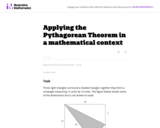Unrestricted Use
CC BY
Rating
0.0 stars

This task requires students to apply the Pythagorean Theorem.

Subject:
Mathematics
Geometry
Material Type:
Activity/Lab
Provider:
Illustrative Mathematics
Provider Set:
Illustrative Mathematics
Author:
Illustrative Mathematics
05/01/2012Unrestricted Use
CC BY
Rating
0.0 stars

Khan Aacdemy provides free resources for teachers and students in various subjects. In the subject of Math, you can videos; tutorials, and practice for your students.

Subject:
Mathematics
Material Type:
Lesson
Author:
Jodi Barnes
08/18/2022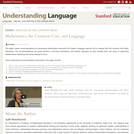Some Rights Reserved
Rating
0.0 stars

This paper makes recommendations for developing mathematics instruction for English Language Learners (ELLs) aligned with the Common Core State Standards. The recommendations can guide teachers, curriculum developers, and teacher educators as they develop their own ways of supporting mathematical reasoning and sense-making for ELLs.Some instructional recommendations discussed in the paper include: Focus on ELL students' mathematical reasoning, not the correctness of their mathematical language use. Shift to a focus on mathematical discourse practices; move away from simplified views of language. Support ELL students as they engage in complex mathematical language. Use ELL students' language and experiences as resources. Provide professional development to enhance teachers' awareness of ways to support ELs as they develop both language and mathematical knowledge.

Subject:
Language Education (ESL)
Material Type:
Teaching/Learning Strategy
Provider:
Stanford University School of Education
Provider Set:
Understanding Language
Author:
Judit Moschkovich
03/02/2012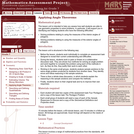Only Sharing Permitted
CC BY-NC-ND
Rating
4.0 stars

This lesson unit is intended to help you assess how well students are able to use geometric properties to solve problems. In particular, it will support you in identifying and helping students who have the following difficulties: Solving problems relating to using the measures of the interior angles of polygons; and solving problems relating to using the measures of the exterior angles of polygons.

Subject:
Geometry
Material Type:
Assessment
Lesson Plan
Provider:
Shell Center for Mathematical Education
Provider Set:
Mathematics Assessment Project (MAP)
04/26/2013Unrestricted Use
CC BY
Rating
5.0 stars

Mathematically Productive Instructional Routines (MPIR) are short (10ish minutes), daily exercises aimed at building number sense. These six different MPIR are part of the Mathematically Productive Instructional Routines collection from the Washington Office of Public Instruction and the Washington Association of Educational Service Districts.

Subject:
Mathematics
Material Type:
Teaching/Learning Strategy
Author:
Barbara Soots
Washington OSPI OER Project
Washington OSPI Mathematics Department
04/05/2021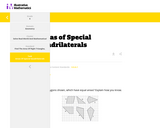Conditional Remix & Share Permitted
CC BY-NC-SA
Rating
0.0 stars

This task asks students to identify which of the six polygons have the same area. Students may complete the task using a variety of techniques including decomposing shapes, using transformations (rotations, reflections, translations) to move one or more parts of the figure to another part to more easily calculate the area, enclosing the polygon inside a larger rectangle and then subtract the areas of the "extra" pieces, etc.

Subject:
Mathematics
Material Type:
Activity/Lab
Diagram/Illustration
Lesson Plan
Provider:
Illustrative Mathematics
05/08/2020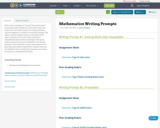Conditional Remix & Share Permitted
CC BY-NC
Rating
0.0 stars

This is three examples of "Type III" documents that I have prepared for students to write about math. Our school uses the Collins system of writing, but these could be adapted to a variety of classroom settings. The topics could be middle school or early high school topics, and they are set up to allow students to indepedently express their knowledge of the given topics in short essay form.

In addition to the writing prompts, I have included a rubric that students use to peer-edit each others' rough drafts. Students then use this feedback form to make the necessary corrections and submit a subsequent final draft.

Subject:
Mathematics
Material Type:
Activity/Lab
Assessment
Author:
Jason Rothgeb
12/04/2023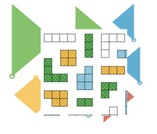Conditional Remix & Share Permitted
CC BY-NC
Rating
0.0 stars

Download GeoGebra Illustrative Mathematics LMS course files. All of GeoGebra's Illustrative Mathematics apps have been placed via links into Moodle courses - each unit is one course. There are twenty save middles school courses and more for high school (the list is growing.) These course files can then be uploaded into Moodle, Schoology, Canvas or D2L.

Subject:
Mathematics
Material Type:
Full Course
Author:
Dan MGuire
Diana Fisher Ph.D.
04/07/2021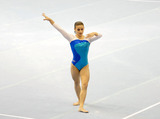Conditional Remix & Share Permitted
CC BY-NC
Rating
0.0 stars

Lesson OverviewStudents apply the multiplication property of equality to solve equations.Key ConceptsIn the previous lesson, students solved equations of the form x + p = q using the addition property of equality. In this lesson, they will solve equations of the form px = q using the multiplication property of equality. They will multiply or divide both sides of an equation by the same number to obtain an equivalent equation.Since multiplication by a is equivalent to division by 1a, students will see that they may also divide both sides of the equation by the same number to get an equivalent equation. Students will also apply this property to solving a particular kind of equation, a proportion.Goals and Learning ObjectivesUse the multiplication property of equality to keep an equation balanced.Use the multiplication property of equality to solve equations of the form px = q for cases in which p, q, and x are all non-negative rational numbers.Use the multiplication property of equality to solve proportions.

Subject:
Algebra
Material Type:
Lesson Plan
09/21/2015Conditional Remix & Share Permitted
CC BY-NC
Rating
0.0 stars

Students review the ways classroom habits and routines can strengthen their mathematical character. Students learn what a Gallery is and how to choose a Gallery problem to work on. They then choose one of three Gallery problems that introduce the unit’s technology resources. The three Gallery problems combine working with expressions with the resources available with this unit.Key ConceptsUnderstand that a Gallery gives students a choice of several problems. Understand what to consider when choosing a problem. Know how to work on a Gallery problem and how to present work on gallery problems.Goals and Learning ObjectivesKnow how to choose a problem from a Gallery.

Subject:
Mathematics
Material Type:
Lesson Plan
09/21/2015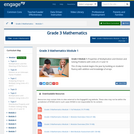Conditional Remix & Share Permitted
CC BY-NC-SA
Rating
0.0 stars

This 25-day module begins the year by building on students fluency with addition and knowledge of arrays.

Find the rest of the EngageNY Mathematics resources at https://archive.org/details/engageny-mathematics.

Subject:
Numbers and Operations
Material Type:
Module
Provider:
New York State Education Department
Provider Set:
EngageNY
05/11/2013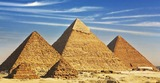Conditional Remix & Share Permitted
CC BY-NC
Rating
0.0 stars

Students discover the formula for finding the volume of a pyramid and apply the formula to solve problems.Key ConceptsThe volume of a pyramid is one-third the volume of a prism with the same base and height. The shape of the base does not matter (including if it’s a circle), and students will see the same one-third comparison between a cylinder and cone.GoalsUnderstand the formula for the volume of a pyramid.Apply the volume formula to solve problems.

Subject:
Geometry
Material Type:
Lesson Plan
09/21/2015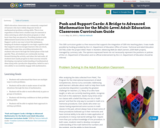Conditional Remix & Share Permitted
CC BY-NC-SA
Rating
5.0 stars

Adult education classrooms are commonly comprised of learners who have widely disparate levels of mathematical problem-solving skills. This is true regardless of what level a student may be assessed at when entering an adult education program or what level class they are placed in. Providing students with differentiated instruction in the form of Push and Support cards is one way to level this imbalance, keeping all students engaged in one high-cognitive task that supports and encourages learners who are stuck, while at the same time, providing extensions for students who move through the initial phase of the task quickly. Thus, all
students are continually moving forward during the activity, and when the task ends, all students have made progress in their journey towards developing conceptual understanding of mathematical ideas along with a productive disposition, belief in one’s own ability to successfully engage with mathematics.

Subject:
Mathematics
Material Type:
Lesson Plan
Module
Teaching/Learning Strategy
Author:
Patricia Helmuth
12/04/2023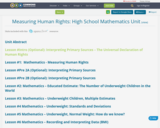Unrestricted Use
CC BY
Rating
5.0 stars

In this unit, students will read and interpret primary sources to address the question “How do we measure the attainment of human rights?” By exploring the Universal Declaration of Human Rights, the UN’s Guide to Indicators of Human Rights, and data about development indicators from multiple databases, students will unpack the complexities of using indicators to measure human rights.

Subject:
Mathematics
Material Type:
Assessment
Lesson Plan
Author:
Tamar Posner
12/04/2023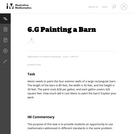Unrestricted Use
CC BY
Rating
0.0 stars

The purpose of this task is to provide students an opportunity to use mathematics addressed in different standards in the same problem.

Subject:
Mathematics
Geometry
Material Type:
Activity/Lab
Provider:
Illustrative Mathematics
Provider Set:
Illustrative Mathematics
Author:
Illustrative Mathematics
05/01/2012Unrestricted Use
CC BY
Rating
0.0 stars

This is a task from the Illustrative Mathematics website that is one part of a complete illustration of the standard to which it is aligned. Each task has at least one solution and some commentary that addresses important aspects of the task and its potential use.

Subject:
Geometry
Material Type:
Activity/Lab
Provider:
Illustrative Mathematics
Provider Set:
Illustrative Mathematics
Author:
Illustrative Mathematics
08/06/2015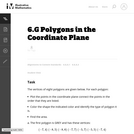Unrestricted Use
CC BY
Rating
0.0 stars

This is a task from the Illustrative Mathematics website that is one part of a complete illustration of the standard to which it is aligned. Each task has at least one solution and some commentary that addresses important asects of the task and its potential use. Here are the first few lines of the commentary for this task: The vertices of eight polygons are given below. For each polygon: * Plot the points in the coordinate plane connect the points in the order that they a...

Subject:
Mathematics
Material Type:
Activity/Lab
Provider:
Illustrative Mathematics
Provider Set:
Illustrative Mathematics
Author:
Illustrative Mathematics
09/08/2013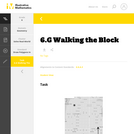Unrestricted Use
CC BY
Rating
0.0 stars

This is a task from the Illustrative Mathematics website that is one part of a complete illustration of the standard to which it is aligned. Each task has at least one solution and some commentary that addresses important aspects of the task and its potential use.

Subject:
Geometry
Material Type:
Activity/Lab
Provider:
Illustrative Mathematics
Provider Set:
Illustrative Mathematics
Author:
Illustrative Mathematics
08/06/2015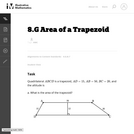Unrestricted Use
CC BY
Rating
0.0 stars

This is a task from the Illustrative Mathematics website that is one part of a complete illustration of the standard to which it is aligned. Each task has at least one solution and some commentary that addresses important asects of the task and its potential use. Here are the first few lines of the commentary for this task: Quadrilateral $ABCD$ is a trapezoid, $AD = 15$, $AB = 50$, $BC = 20$, and the altitude is 12. What is the area of the trapezoid?...

Subject:
Mathematics
Material Type:
Activity/Lab
Provider:
Illustrative Mathematics
Provider Set:
Illustrative Mathematics
Author:
Illustrative Mathematics
03/17/2013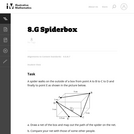Unrestricted Use
CC BY
Rating
0.0 stars

This is a task from the Illustrative Mathematics website that is one part of a complete illustration of the standard to which it is aligned. Each task has at least one solution and some commentary that addresses important asects of the task and its potential use. Here are the first few lines of the commentary for this task: A spider walks on the outside of a box from point A to B to C to D and finally to point E as shown in the picture below. Draw a net of the box and map ...

Subject:
Mathematics
Material Type:
Activity/Lab
Provider:
Illustrative Mathematics
Provider Set:
Illustrative Mathematics
Author:
Illustrative Mathematics
11/25/2013Unrestricted Use
CC BY
Rating
0.0 stars

This is a task from the Illustrative Mathematics website that is one part of a complete illustration of the standard to which it is aligned. Each task has at least one solution and some commentary that addresses important asects of the task and its potential use. Here are the first few lines of the commentary for this task: Let $A$ be the area of a triangle with sides of length 25, 25, and 30. Let $B$ be the area of a triangle with sides of length 25, 25, and 40. Find A/B... Subject: Mathematics Material Type: Activity/Lab Provider: Illustrative Mathematics Provider Set: Illustrative Mathematics Author: Illustrative Mathematics Date Added: 05/29/2013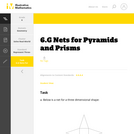Unrestricted Use CC BY Rating 0.0 stars This is a task from the Illustrative Mathematics website that is one part of a complete illustration of the standard to which it is aligned. Each task has at least one solution and some commentary that addresses important aspects of the task and its potential use. Subject: Geometry Material Type: Activity/Lab Provider: Illustrative Mathematics Provider Set: Illustrative Mathematics Author: Illustrative Mathematics Date Added: 08/06/2015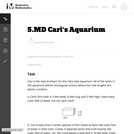Unrestricted Use CC BY Rating 0.0 stars This is a task from the Illustrative Mathematics website that is one part of a complete illustration of the standard to which it is aligned. Each task has at least one solution and some commentary that addresses important asects of the task and its potential use. Here are the first few lines of the commentary for this task: Cari is the lead architect for the city’s new aquarium. All of the tanks in the aquarium will be rectangular prisms where the side lengths are whole nu... Subject: Mathematics Material Type: Activity/Lab Provider: Illustrative Mathematics Provider Set: Illustrative Mathematics Author: Illustrative Mathematics Date Added: 05/13/2013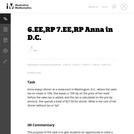Unrestricted Use CC BY Rating 0.0 stars This is a task from the Illustrative Mathematics website that is one part of a complete illustration of the standard to which it is aligned. Each task has at least one solution and some commentary that addresses important asects of the task and its potential use. Here are the first few lines of the commentary for this task: Anna enjoys dinner at a restaurant in Washington, D.C., where the sales tax on meals is 10%. She leaves a 15% tip on the price of her meal before the s... Subject: Mathematics Material Type: Activity/Lab Provider: Illustrative Mathematics Provider Set: Illustrative Mathematics Author: Illustrative Mathematics Date Added: 03/17/2013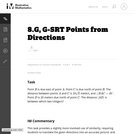Unrestricted Use CC BY Rating 0.0 stars This is a task from the Illustrative Mathematics website that is one part of a complete illustration of the standard to which it is aligned. Each task has at least one solution and some commentary that addresses important asects of the task and its potential use. Here are the first few lines of the commentary for this task: PointB$is due east of point$A$. Point$C$is due north of point$B$. The distance between points$A$and$C$is$10\sqrt 2$meters, and$\angle BAC...

Subject:
Mathematics
Material Type:
Activity/Lab
Provider:
Illustrative Mathematics
Provider Set:
Illustrative Mathematics
Author:
Illustrative Mathematics
03/29/2013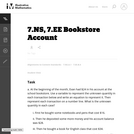Unrestricted Use
CC BY
Rating
0.0 stars

This is a task from the Illustrative Mathematics website that is one part of a complete illustration of the standard to which it is aligned. Each task has at least one solution and some commentary that addresses important asects of the task and its potential use. Here are the first few lines of the commentary for this task: At the beginning of the month, Evan had \24 in his account at the school bookstore. Use a variable to represent the unknown quantity in each transacti... Subject: Mathematics Material Type: Activity/Lab Provider: Illustrative Mathematics Provider Set: Illustrative Mathematics Author: Illustrative Mathematics Date Added: 05/13/2013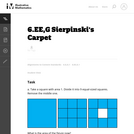Unrestricted Use CC BY Rating 0.0 stars This is a task from the Illustrative Mathematics website that is one part of a complete illustration of the standard to which it is aligned. Each task has at least one solution and some commentary that addresses important asects of the task and its potential use. Here are the first few lines of the commentary for this task: Take a square with area 1. Divide it into 9 equal-sized squares. Remove the middle one. What is the area of the figure now? Take the remaining 8 square... Subject: Mathematics Material Type: Activity/Lab Provider: Illustrative Mathematics Provider Set: Illustrative Mathematics Author: Illustrative Mathematics Date Added: 08/06/2013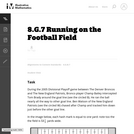Unrestricted Use CC BY Rating 0.0 stars This is a task from the Illustrative Mathematics website that is one part of a complete illustration of the standard to which it is aligned. Each task has at least one solution and some commentary that addresses important asects of the task and its potential use. Here are the first few lines of the commentary for this task: During the 2005 Divisional Playoff game between The Denver Broncos and The New England Patriots, Bronco player Champ Bailey intercepted Tom Brady aroun... Subject: Mathematics Material Type: Activity/Lab Provider: Illustrative Mathematics Provider Set: Illustrative Mathematics Author: Illustrative Mathematics Date Added: 03/17/2013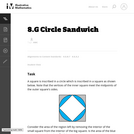Unrestricted Use CC BY Rating 0.0 stars This is a task from the Illustrative Mathematics website that is one part of a complete illustration of the standard to which it is aligned. Each task has at least one solution and some commentary that addresses important asects of the task and its potential use. Here are the first few lines of the commentary for this task: A square is inscribed in a circle which is inscribed in a square as shown below. Note that the vertices of the inner square meet the midpoints of the o... Subject: Mathematics Material Type: Activity/Lab Provider: Illustrative Mathematics Provider Set: Illustrative Mathematics Author: Illustrative Mathematics Date Added: 09/08/2013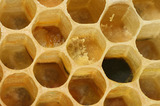Conditional Remix & Share Permitted CC BY-NC Rating 0.0 stars Students will resume their project and decide on dimensions for their buildings. They will use scale to calculate the dimensions and areas of their model buildings when full size. Students will also complete a Self Check in preparation for the Putting It Together lesson.Key ConceptsThe first part of the project is essentially a review of the unit so far. Students will find the area of a composite figure—either a polygon that can be broken down into known areas, or a regular polygon. Students will also draw the figure using scale and find actual lengths and areas.GoalsRedraw a scale drawing at a different scale.Find measurements using a scale drawing.Find the area of a composite figure.SWD: Consider what supplementary materials may benefit and support students with disabilities as they work on this project:Vocabulary resource(s) that students can reference as they work:List of formulas, with visual supports if appropriateClass summaries or lesson artifacts that help students to recall and apply newly introduced skillsChecklists of expectations and steps required to promote self-monitoring and engagementModels and examplesStudents with disabilities may take longer to develop a solid understanding of newly introduced skills and concepts. They may continue to require direct instruction and guided practice with the skills and concepts relating to finding area and creating and interpreting scale drawings. Check in with students to assess their understanding of newly introduced concepts and plan review and reinforcement of skills as needed.ELL: As academic vocabulary is reviewed, be sure to repeat it and allow students to repeat after you as needed. Consider writing the words as they are being reviewed. Allow enough time for ELLs to check their dictionaries if they wish. Subject: Geometry Material Type: Lesson Plan Date Added: 09/21/2015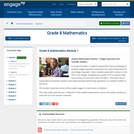Conditional Remix & Share Permitted CC BY-NC-SA Rating 0.0 stars In Grade 8 Module 1, students expand their basic knowledge of positive integer exponents and prove the Laws of Exponents for any integer exponent. Next, students work with numbers in the form of an integer multiplied by a power of 10 to express how many times as much one is than the other. This leads into an explanation of scientific notation and continued work performing operations on numbers written in this form. Find the rest of the EngageNY Mathematics resources at https://archive.org/details/engageny-mathematics. Subject: Numbers and Operations Material Type: Module Provider: New York State Education Department Provider Set: EngageNY Date Added: 05/14/2013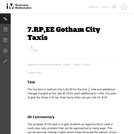Unrestricted Use CC BY Rating 0.0 stars This is a task from the Illustrative Mathematics website that is one part of a complete illustration of the standard to which it is aligned. Each task has at least one solution and some commentary that addresses important asects of the task and its potential use. Here are the first few lines of the commentary for this task: The taxi fare in Gotham City is \2.40 for the first $\frac{1}{2}$ mile and additional mileage charged at the rate \\$0.20 for each additional 0.1 mile....

Subject:
Mathematics
Material Type:
Activity/Lab
Provider:
Illustrative Mathematics
Provider Set:
Illustrative Mathematics
Author:
Illustrative Mathematics
03/17/2013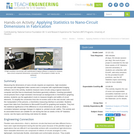Educational Use
Rating
0.0 stars

Measuring the dimensions of nano-circuits requires an expensive, high-resolution microscope with integrated video camera and a computer with sophisticated imaging software, but in this activity, students measure nano-circuits using a typical classroom computer and (the free-to-download) GeoGebra geometry software. Inserting (provided) circuit pictures from a high-resolution microscope as backgrounds in GeoGebra's graphing window, students use the application's tools to measure lengths and widths of circuit elements. To simplify the conversion from the on-screen units to the real circuits' units and the manipulation of the pictures, a GeoGebra measuring interface is provided. Students export their data from GeoGebra to Microsoft® Excel® for graphing and analysis. They test the statistical significance of the difference in circuit dimensions, as well as obtain a correlation between average changes in original vs. printed circuits' widths. This activity and its associated lesson are suitable for use during the last six weeks of the AP Statistics course; see the topics and timing note below for details.

Subject:
Mathematics
Statistics and Probability
Material Type:
Activity/Lab
Provider:
TeachEngineering
Provider Set:
TeachEngineering
Author:
Cunjiang Yu
Miguel R. Ramirez
Minwei Xu
Song Chen
02/17/2017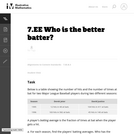Unrestricted Use
CC BY
Rating
0.0 stars

This is a task from the Illustrative Mathematics website that is one part of a complete illustration of the standard to which it is aligned. Each task has at least one solution and some commentary that addresses important asects of the task and its potential use. Here are the first few lines of the commentary for this task: Below is a table showing the number of hits and the number of times at bat for two Major League Baseball players during two different seasons: SeasonDe...

Subject:
Mathematics
Material Type:
Activity/Lab
Provider:
Illustrative Mathematics
Provider Set:
Illustrative Mathematics
Author:
Illustrative Mathematics
08/10/2013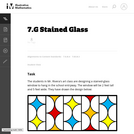Unrestricted Use
CC BY
Rating
0.0 stars

This is a task from the Illustrative Mathematics website that is one part of a complete illustration of the standard to which it is aligned. Each task has at least one solution and some commentary that addresses important asects of the task and its potential use. Here are the first few lines of the commentary for this task: The students in Mr. Rivera's art class are designing a stained-glass window to hang in the school entryway. The window will be 2 feet tall and 5 feet w...

Subject:
Mathematics
Material Type:
Activity/Lab
Provider:
Illustrative Mathematics
Provider Set:
Illustrative Mathematics
Author:
Illustrative Mathematics
08/19/2013Unrestricted Use
CC BY
Rating
0.0 stars

This purpose of this task is to help students see two different ways to look at percentages both as a decrease and an increase of an original amount. In addition, students have to turn a verbal description of several operations into mathematical symbols.

Subject:
Mathematics
Algebra
Material Type:
Activity/Lab
Provider:
Illustrative Mathematics
Provider Set:
Illustrative Mathematics
Author:
Illustrative Mathematics
05/01/2012Conditional Remix & Share Permitted
CC BY-NC
Rating
0.0 stars

Math in Real Life (MiRL) supports the expansion of regional networks to create an environment of innovation in math teaching and learning.  The focus on applied mathematics supports the natural interconnectedness of math to other disciplines while infusing relevance for students.  MiRL supports a limited number of networked math learning communities that focus on developing and testing applied problems in mathematics.  The networks help math teachers refine innovative teaching strategies with the guidance of regional partners and the Oregon Department of Education.

Subject:
Mathematics
Material Type:
Homework/Assignment
Author:
Mark Freed
Tom Thompson
07/27/2020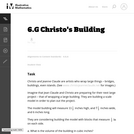Unrestricted Use
CC BY
Rating
0.0 stars

This task is primarily about volume and surface area, although it also gives students an early look at converting between measurements in scale models and the real objects they correspond to.

Subject:
Mathematics
Geometry
Material Type:
Activity/Lab
Provider:
Illustrative Mathematics
Provider Set:
Illustrative Mathematics
Author:
Illustrative Mathematics
05/01/2012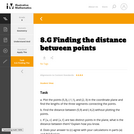Unrestricted Use
CC BY
Rating
0.0 stars

This is a task from the Illustrative Mathematics website that is one part of a complete illustration of the standard to which it is aligned. Each task has at least one solution and some commentary that addresses important aspects of the task and its potential use.

Subject:
Geometry
Material Type:
Activity/Lab
Provider:
Illustrative Mathematics
Provider Set:
Illustrative Mathematics
Author:
Illustrative Mathematics
08/06/2015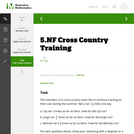Unrestricted Use
CC BY
Rating
0.0 stars

This is a task from the Illustrative Mathematics website that is one part of a complete illustration of the standard to which it is aligned. Each task has at least one solution and some commentary that addresses important aspects of the task and its potential use.

Subject:
Ratios and Proportions
Material Type:
Activity/Lab
Provider:
Illustrative Mathematics
Provider Set:
Illustrative Mathematics
Author:
Illustrative Mathematics